Want to share your content on R-bloggers? click here if you have a blog, or here if you don't.

In the second part of my “how to quickly visualize networks directly from R” series, I’ll discuss how to use R and the “rgexf package to create network plots in Gephi. Gephi is a great network visualization tool that allows real-time network visualization and exploration, including network data spatializing, filtering, calculation of network properties, and clustering. Unfortunately, a current version of the “rgexf” package does not support real-time network visualization and exploration. However, it allows users to create a .gexf file that contains information about network nodes and edges (and their properties); such file can be loaded and visualized by Gephi.

To demonstrate how this works, I will use the same network I used to demonstrate network visualization in Cytoscape – the weighted network of characters’ coappearances in Victor Hugo’s novel “Les Miserables” (LesMiserables.txt). I will also use the same node and edge properties: the degree of a node, betweenness centrality of a node, Dice similarity of two nodes, and the coappearance weight. The idea is to use “rgexf” to create a network visualization in Gephi that captures the network patterns in the similar way the network visualization in “RCytoscape”/Cytoscape did. For more information, see Network visualization – part 1). See the bottom of this post for full code.

To create a .gexf file, I used “write.gexf” function. This function creates a gexf representation of a network, based on a user specified data frames describing network nodes and edges. Given the Les Miserables network in the “igraph” format (denoted as “gD”), we can easily create a data frame describing network nodes as:

`data.frame(ID = c(1:vcount(gD)), NAME = V(gD)\$name)`

and data frame describing network edges as:

`as.data.frame(get.edges(gD, c(1:ecount(gD))))`

“write.gexf” also allows users to assign any type of node and edge attributes to nodes and edges in the network. If we decide to use node/edge attributes, we have to define each attribute for all nodes/edges and the order of attribute values has to correspond to the order of nodes/edges in the edge/node data frames.

We assigned the degree and the betweenness centrality to nodes in our network as:

`data.frame(DEG = V(gD)\$degree, BET = V(gD)\$betweenness)`

Similarly, we assigned the Dice similarity the co-appearance weight to edges in our network as:

`data.frame(WGH = E(gD)\$weight, SIM = E(gD)\$similarity)`

This was easy.

In addition to these (basic) options, the “write.gexf” function provides options to define node and edge visualization attributes, e.g., their color, position, and size/thickness, type of the network (directed or undirected), as well as the edge dynamics. Here, I‘ll focus only on visualization options.

Some of the available visualization attributes in “write.gexf” are not supported by the current version of Gephi, e.g., different node and edge shapes within the same network or representation of nodes as images (although, it is great that the “rgexf” authors thought ahead).

First, let’s define node coordinates. The easiest way to do so is with the “igraph” function “layout.” As my goal is to create as similar network plot as I did in Cytoscape, I used a Fruchterman and Reingold’s force-based layout algorithm. Similarly as before, I used the Dice similarity to define the force between two nodes. The “layout.fruchterman.reingold” function can output node layout/coordinates in 2D and 3D. The “write.gexf” function requires that node coordinates are 3D (another think ahead option?). However, Gephi plots networks in 2D and using 3D coordinates for 2D plots will not result in the nicest layout/plot. For that reason, I decided to cheat – create 2D layout/coordinates, and assign 0 as the third coordinate.

Next, I defined node size. To stay consistent with the Cytoscape plot, node size should be interpolated based on the node betweenness centrality. As the “rgexf” library does not provide any options for node size/color interpolation, I had to do it myself. I defined the node size interval as [1, 5] (thus,  min node size is 1, max node size is 5). I used the “approx” function to interpolate the node size among n unique betweenness values:

`approx(c(1, 5), n = length(unique(V(gD)\$betweenness)))`

Then, I assigned the interpolated node size values to each node as:

```sapply(V(gD)\$betweenness, function(x) approxVals\$y[which(sort(unique(V(gD)\$betweenness)) == x)])
```

Note that this this approach scales node size values solely based on the number of unique betweenness centrality values, not based on the ratio of those values.

Similarly, for node color, I needed to define a way to interpolate node colors based on the node degree. To do so, I used the “colorRampPalette” function from the “grDevices” library:

```F2 <- colorRampPalette(c("#F5DEB3", "#FF0000"),
bias = length(unique(V(gD)\$degree)),
space = "rgb", interpolate = "linear")```

This function returns another function (I called it F2), that allows us to create a color palette. Next, we assign a color for each of the degree values:

`colCodes <- F2(length(unique(V(gD)\$degree)))`

Finally, similarly as before, we used the “sapply” function to map colors to the corresponding nodes:

```sapply(V(gD)\$degree,
function(x) colCodes[which(sort(unique(V(gD)\$degree)) == x)])
```

“write.gexf” requires that node colors are presented in the RGBA color mode, i.e., with a 4 coordinates: r, g, b, and alpha; r, g, b are integers between 0 and 256, and alpha is a float between 0.0 and 1.0, corresponding to node transparency. Currently, our colors are in RGB hexadecimal and  we need to transform them to RGB decimal. To do so, I’ll use the “col2rgb” function:

`col2rgb(nodes_col, alpha = FALSE)`

Although this function can return the alpha value, the value it returns is in [0, 255] and does not match the alpha value required by “write.gexf.” For that reason, I manually assigned alpha = 1 to all node.

With this we defined all node visual attributes. Similar strategy can be applied to define edge visual attributes and save/write the network. The example of the .gexf network  made in this example is available in “lesmis.gexf” file. So, let’s see how this network looks in Gephi.

When opening the .gexf file, be sure to deselect “Auto-scale” and “Create missing nodes” options in the Gephi Import report. Also, it seems that the “defaultedgetype ” options written in the .gexf file was not recognized, so change the “Graph Type” to “Undirected.”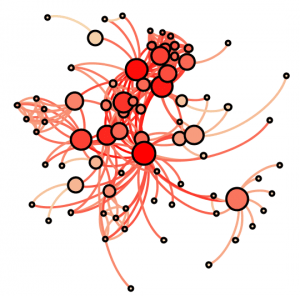We can see that the network layout fits the force based layout, and that the node size and colors correspond to node properties. However, edge colors do not match those we specified. This does not mean that our color attributes do not exist, but that they were not selected by default. To change this, we have to go to “Preview settings” -> “Edges” -> “Colors” and select “Original.”

And here is our network.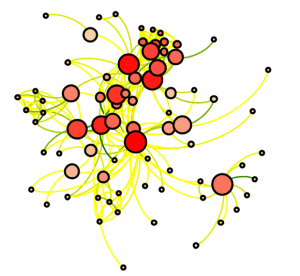To show node names, we have to go to “Preview settings” -> “Node labels” and check the box “Show labels.” We can scale the labels sizes (to match node sizes) if we check box “Proportional size.” Here is the final version of our network plot.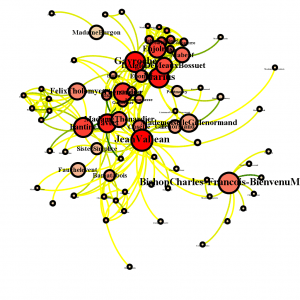Although the plot shows patterns in node connectivity, many nodes and labels are overlapping with each other. It is clear that in order to get a high-quality plot, we need to make additional adjustments within the Gephi. This raises a question of whether or not user specified visual attributes are an efficient way to define network visual characteristics and how successfully “write.gexf“ can translate these attributes into a user specified network plot.

To explore this question, I created a network using only information about network, nodes, edges, and their attributes (thus, no visualization attributes). Then, I used Gephi network visualization options (within Gephi) to create layout and adjust node/edge attributes. In about the same number of steps as it took me to “show”  the visual attributes in the previous step, I was able to create the following network plot.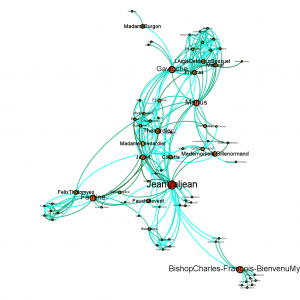This network is clearly more appealing than the one generated based on the visual attributes specified in the .gexf file. This implies that there is almost no gain in defining user specified visualization attributes in the current version of “rgexf”/”write.gexf,” as the user-specified visualization .gexf network file does not results in “ready-to-use” plots.

If you ever used Gephi you probably noticed that the “Les Miserables” network is one of the network examples that come with Gephi. Here is the Les Miserables network that shows all the visualization power of Gephi. Hopefully, soon we’ll be able to create the same quality network plots directly from R.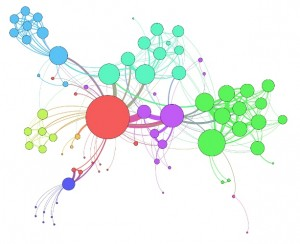Here is the complete code used for the Gephi visualization example.

```# Plotting networks in R
# An example how to use R and rgexf package to create a .gexf file for network visualization in Gephi

############################################################################################
# Clear workspace
rm(list = ls())

library("igraph")
library("plyr")

# Data format: dataframe with 3 variables; variables 1 & 2 correspond to interactions; variable 3 corresponds to the weight of interaction

# Create a graph. Use simplify to ensure that there are no duplicated edges or self loops
gD <- simplify(graph.data.frame(dataSet, directed=FALSE))

# Print number of nodes and edges
# vcount(gD)
# ecount(gD)

############################################################################################
# Calculate some node properties and node similarities that will be used to illustrate
# different plotting abilities

# Calculate degree for all nodes
degAll <- degree(gD, v = V(gD), mode = "all")

# Calculate betweenness for all nodes
betAll <- betweenness(gD, v = V(gD), directed = FALSE) / (((vcount(gD) - 1) * (vcount(gD)-2)) / 2)
betAll.norm <- (betAll - min(betAll))/(max(betAll) - min(betAll))
rm(betAll)

# Calculate Dice similarities between all pairs of nodes
dsAll <- similarity.dice(gD, vids = V(gD), mode = "all")

############################################################################################
# Add new node/edge attributes based on the calculated node properties/similarities

gD <- set.vertex.attribute(gD, "degree", index = V(gD), value = degAll)
gD <- set.vertex.attribute(gD, "betweenness", index = V(gD), value = betAll.norm)

# Check the attributes
# summary(gD)

F1 <- function(x) {data.frame(V4 = dsAll[which(V(gD)\$name == as.character(x\$V1)), which(V(gD)\$name == as.character(x\$V2))])}
dataSet.ext <- ddply(dataSet, .variables=c("V1", "V2", "V3"), function(x) data.frame(F1(x)))

gD <- set.edge.attribute(gD, "weight", index = E(gD), value = 0)
gD <- set.edge.attribute(gD, "similarity", index = E(gD), value = 0)

# The order of interactions in gD is not the same as it is in dataSet or as it is in the edge list,
# and for that reason these values cannot be assigned directly

E(gD)[as.character(dataSet.ext\$V1) %--% as.character(dataSet.ext\$V2)]\$weight <- as.numeric(dataSet.ext\$V3)
E(gD)[as.character(dataSet.ext\$V1) %--% as.character(dataSet.ext\$V2)]\$similarity <- as.numeric(dataSet.ext\$V4)

# Check the attributes
# summary(gD)

####################################
# Print network in the file format ready for Gephi
# This requires rgexf package

library("rgexf")

# Create a dataframe nodes: 1st column - node ID, 2nd column -node name
nodes_df <- data.frame(ID = c(1:vcount(gD)), NAME = V(gD)\$name)
# Create a dataframe edges: 1st column - source node ID, 2nd column -target node ID
edges_df <- as.data.frame(get.edges(gD, c(1:ecount(gD))))

# Define node and edge attributes - these attributes won't be directly used for network visualization, but they
# may be useful for other network manipulations in Gephi
#
# Create a dataframe with node attributes: 1st column - attribute 1 (degree), 2nd column - attribute 2 (betweenness)
nodes_att <- data.frame(DEG = V(gD)\$degree, BET = V(gD)\$betweenness)
#
# Create a dataframe with edge attributes: 1st column - attribute 1 (weight), 2nd column - attribute 2 (similarity)
edges_att <- data.frame(WGH = E(gD)\$weight, SIM = E(gD)\$similarity)

# Define node/edge visual attributes - these attributes are the ones used for network visualization
#
# Calculate node coordinate - needs to be 3D
#nodes_coord <- as.data.frame(layout.fruchterman.reingold(gD, weights = E(gD)\$similarity, dim = 3, niter = 10000))
# We'll cheat here, as 2D coordinates result in a better (2D) plot than 3D coordinates
nodes_coord <- as.data.frame(layout.fruchterman.reingold(gD, weights = E(gD)\$similarity, dim = 2, niter = 10000))
nodes_coord <- cbind(nodes_coord, rep(0, times = nrow(nodes_coord)))
#
# Calculate node size
# We'll interpolate node size based on the node betweenness centrality, using the "approx" function
approxVals <- approx(c(1, 5), n = length(unique(V(gD)\$betweenness)))
# And we will assign a node size for each node based on its betweenness centrality
nodes_size <- sapply(V(gD)\$betweenness, function(x) approxVals\$y[which(sort(unique(V(gD)\$betweenness)) == x)])
#
# Define node color
# We'll interpolate node colors based on the node degree using the "colorRampPalette" function from the "grDevices" library
library("grDevices")
# This function returns a function corresponding to a collor palete of "bias" number of elements
F2 <- colorRampPalette(c("#F5DEB3", "#FF0000"), bias = length(unique(V(gD)\$degree)), space = "rgb", interpolate = "linear")
# Now we'll create a color for each degree
colCodes <- F2(length(unique(V(gD)\$degree)))
# And we will assign a color for each node based on its degree
nodes_col <- sapply(V(gD)\$degree, function(x) colCodes[which(sort(unique(V(gD)\$degree)) == x)])
# Transform it into a data frame (we have to transpose it first)
nodes_col_df <- as.data.frame(t(col2rgb(nodes_col, alpha = FALSE)))
# And add alpha (between 0 and 1). The alpha from "col2rgb" function takes values from 0-255, so we cannot use it
nodes_col_df <- cbind(nodes_col_df, alpha = rep(1, times = nrow(nodes_col_df)))
# Assign visual attributes to nodes (colors have to be 4dimensional - RGBA)
nodes_att_viz <- list(color = nodes_col_df, position = nodes_coord, size = nodes_size)

# Assign visual attributes to edges using the same approach as we did for nodes
F2 <- colorRampPalette(c("#FFFF00", "#006400"), bias = length(unique(E(gD)\$weight)), space = "rgb", interpolate = "linear")
colCodes <- F2(length(unique(E(gD)\$weight)))
edges_col <- sapply(E(gD)\$weight, function(x) colCodes[which(sort(unique(E(gD)\$weight)) == x)])
edges_col_df <- as.data.frame(t(col2rgb(edges_col, alpha = FALSE)))
edges_col_df <- cbind(edges_col_df, alpha = rep(1, times = nrow(edges_col_df)))
edges_att_viz <-list(color = edges_col_df)

# Write the network into a gexf (Gephi) file
#write.gexf(nodes = nodes_df, edges = edges_df, nodesAtt = nodes_att, edgesWeight = E(gD)\$weight, edgesAtt = edges_att, nodesVizAtt = nodes_att_viz, edgesVizAtt = edges_att_viz, defaultedgetype = "undirected", output = "lesmis.gexf")
# And without edge weights
write.gexf(nodes = nodes_df, edges = edges_df, nodesAtt = nodes_att, edgesAtt = edges_att, nodesVizAtt = nodes_att_viz, edgesVizAtt = edges_att_viz, defaultedgetype = "undirected", output = "lesmis.gexf")
```